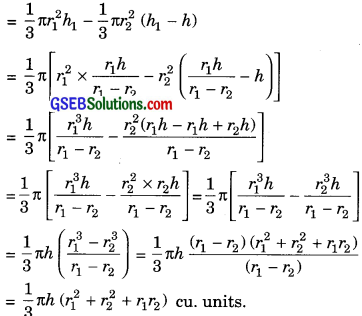# GSEB Solutions Class 10 Maths Chapter 13 Surface Areas and Volumes Ex 13.5

Gujarat Board GSEB Solutions Class 10 Maths Chapter 13 Surface Areas and Volumes Ex 13.5 Textbook Questions and Answers.

## Gujarat Board Textbook Solutions Class 10 Maths Chapter 13 Surface Areas and Volumes Ex 13.5

Question 1.
A copper wire, 3 mm in diameter, is wound about a cylinder whose length is 12 cm, and diameter 10 cm, so as to cover the curved surface of the cylinder. Find the length and mass of the wire, assuming the density of copper to be 8.88 g per cm3.
Solution:
In case of cylinder
Diameter = 10 cm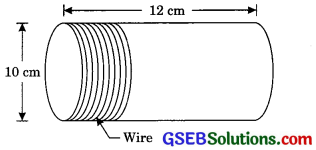Diameter of wire which is rounded over cylinder = 3 mm
Length of cylinder = 12 cm
= 12 x 10 mm = 120 mm
Number of round to cover 120 mm
= $$\frac {120}{3}$$ = 40
Length of wire in completing one round over the cylinder = 2πr
= 2π x 5 = 10π cm
Length of wire in covering whole surface of the cylinder = Length of wire in completing 40 rounds
= 10 π x 40
= 400 π cm
= 400 x 3.14 = 1256 cm
Radius of copper wire = $$\frac {3}{2}$$mm
r1 = $$\frac {3}{20}$$cm
Volume of wire = πr22h
= π ($$\frac {3}{20}$$) x 400n = 9π2 cm3
Mass of wire = 9 π2 x 8.88
= 9 x (3.14)2 x 8.88 g
= 787.98g ≈ 788gQuestion 2.
A right triangle, whose sides are 3 cm and 4 cm (other than hypotenuse) is made to revolve about its hypotenuse. Find the volume and surface area of the double cone so formed. (Choose value of n as found appropriate.)
Solution:
Here
ABC is a right triangle in ∠BAC = 90°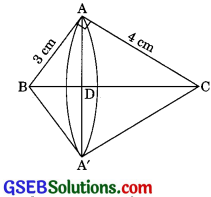BC2 = AB2 + AC2
= 32 + 42 = 9 + 16
BC2 = 25
BC = 5 cm
Here AD and A’D are radii of two cones which are formed by rotating the right triangle about the hypotenuse BC.
ΔADB – ΔCAB (by AA similarity)
$$\frac {AD}{AC}$$ = $$\frac {AB}{BC}$$
⇒ $$\frac {AD}{4}$$ = $$\frac {3}{5}$$
Also $$\frac {BD}{AB}$$ = $$\frac {AB}{BC}$$
$$\frac {BD}{3}$$ = $$\frac {3}{5}$$
⇒ BD = $$\frac {3}{5}$$ cm (h1 = BD say)
Hence CD = 5 – $$\frac {9}{5}$$
h2 = $$\frac {16}{5}$$cm (h2 CD say)
Volume of double cones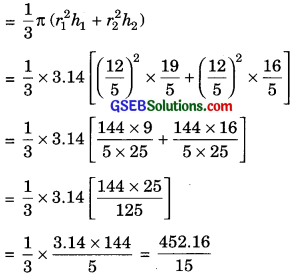And surface area of the double cones
= πr1l1 + πr2l2 = π (r1l1 + r2l2)
= 3.14$$\left[\frac{12}{5} \times 3+\frac{12}{5} \times 4\right]$$ [l1 = 3cm , l2 = 4cm ]
= 3.14 x $$\frac {12}{5}$$ [3 + 4]
= $$\frac{3.14 \times 12 \times 7}{5}$$ = 52.75 cm2Question 3.
A cistern, internally measuring 150 cm x 120 cm x 110 cm, has 129600 cm3 of water in it. Porous bricks are placed in the water until the cistern is full to the brim. Each brick absorbs one-seventeenth of its own volume of water. How many bricks can be put in without overflowing the water, each brick being 22.5 cm x 7.5 cm x 6.5 cm?
Solution:
Volume of cistern = l x b x h
= 150 x 120 x 110
= 1980000 cm3
Volume of water in cistern = 129600 cm3
Volume of cistern to be filled
= Volume of cistern – Volume of water
= 1980000 – 129600
= 1850400 cm3
Let n bricks are needed to fill the cistern up to the brim.
Volume of one brick
= l x b x h
= 22.5 x 7.5 x 6.5
= 1096.875 cm3
Water absorbed by one brick = $$\frac{1096.875}{17}$$ cm3
Water absorbed by n brick = $$\frac{n(1096.875)}{17}$$ cm3
According to the question,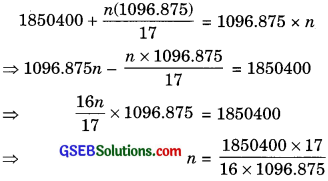n = 1792.41
Hence number of bricks cannot be put in the cistern = 1792

Question 4.
In one fortnight of a given month, there was a rainfall of 10 cm in a river valley. If the area of the valley is 7280 km2, show that the total rainfall was approximately equivalent to the addition to the normal water of three rivers and each 1072 km long, 75 m wide and 3 m deep.
Solution:
Area of valley = 97280 km2
Rainfall = 10 cm
= $$\frac {10}{100}$$ metre = $$\frac{10}{100 \times 1000}$$ km
Volume of rainfall = Area of river valley x Rainfall
= 7280 x $$\frac{10}{100 \times 1000}$$
= 0.728 km3
Volume of three river = 3l x b x h
= 3 x 1072 x $$\frac {75}{1000}$$ x $$\frac {3}{1000}$$
= 0.7236 km3
Hence, total rainfall and normal water of three rivers are approximately equivalent.Question 5.
An oil funnel made of tin sheet consists of a 10 cm long cylindrical portion attached to a frustum of a cone. If the total height is 22 cm, diameter of the cylindrical portion is 8 cm and the diameter of the top of the funnel is 18 cm, find the area of the tin sheet required to make the funnel (See figure).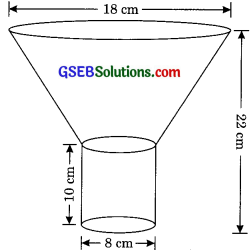Solution:
Here mease of frustum
r1 = $$\frac {18}{2}$$ = 9 cm
r1 = $$\frac {8}{2}$$ = 4 cm
h = 22 – 10 = 12 cm
Slant height of frustum
l = $$\sqrt{\left(r_{1}-r_{2}\right)^{2}+h^{2}}$$
=$$\sqrt{(9-4)^{2}+12^{2}}$$ = $$\sqrt{5^{2}+12^{2}}$$
= $$\sqrt{25+144}$$ = $$\sqrt{169}$$ = 13cm
Area of tin sheet required = Curved surface area of the cylindrical portion + Curved surface area of the frustum portion
= 2πr2h2 + π(r1 + r2)l
= 2 x π x 4 x 10 + π(4 + 9) x 13
= 80π x 169π = 249π
= 249 x $$\frac{22}{7}$$ = $$\frac{5478}{7}$$ = 782 $$\frac{4}{7}$$ cm2Question 6.
Derive the formula for the curved surface area and total surface area of the frustum of a cone, given to you in Section 13.5, using the symbols are explained.
Solution:
Let r1 and r2 be the radii of circular base, where r1 > r2 and h be the height of the frustum of a cone.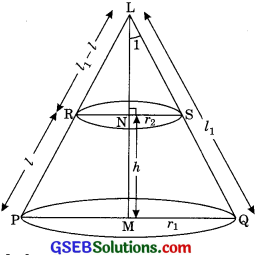Let h1 be the height of large cone LPQ
Height of small cone = h1 – h
Let l1 be the slant height of cone LPQ and l be the frustum of cone. The slant height of Cone LRS
= l1 – l
In rt. ΔLMQ and rt. ΔLNS
∠1 = ∠1 (common)
∠M = ∠N (each 900)
∴ ΔLMQ – ΔLNS (by AA similarity)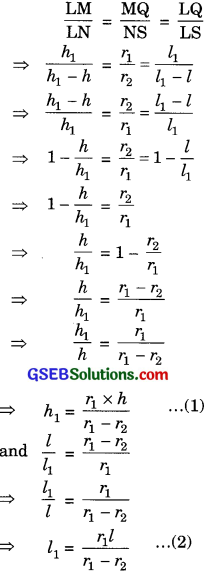(i) Lateral surface area or curved surface area of a frustum of cone = CSA of large cone – CSA of small cone
= πr1l1 – πr2(l1 – l)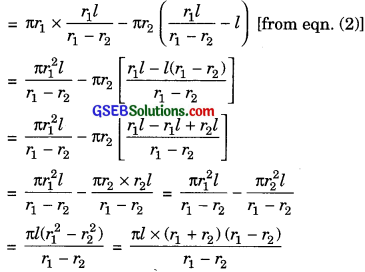= πl(r1 + r2) = π(r1 + r2)l sq. units
(ii) Slant height = $$\sqrt{\left(r_{1}-r_{2}\right)^{2}+h^{2}}$$
(iii) Total surface area of frustum of a cone + πr1r2 + πr2r2
= CSA of frustum of cone + πr21 + πr22
= π(r1 + r2)l + πr21 + πr22 sq. unitsQuestion 7.
Derive the formula for the volume of the frustum of a cone, given to you in Section 13.5, using the symbols as explained.
Solution:
Let r1 and r2 be radii of two circular bases where r1 > r2, h be the height of frustum of a cone.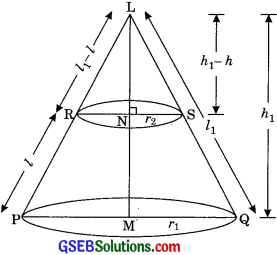Let h1 be the height of large cone LPD and height of small cone LRS = h1 – h
Let l1, be the slant height of cone and t be the slant height of frustum of a cone.
Slant height of cone LRS = l1 – l
In rt. ΔLMQ and rt. ΔLNS (Common)
∠M = ∠N (Each 900)
ΔLMN – ΔLNS (By AA similarity)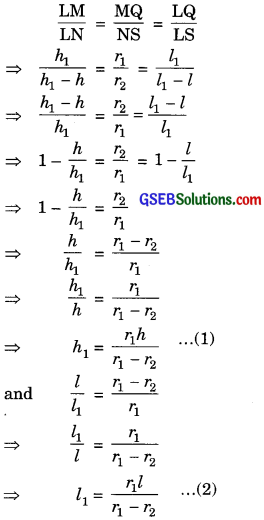Volume of a frustum of a cone = Volume of large cone LPQ – Volume of small cone LRS